[BACK]
 Intelligent Automation & Soft ComputingDOI:10.32604/iasc.2022.022728Article

Fuzzy Based MPPT and Solar Power Forecasting Using Artificial Intelligence

1RMK College of Engineering and Technology, Chennai, 601206, India
2RMK Engineering College, Chennai, 601206, India
*Corresponding Author: G. Geethamahalakshmi. Email: geethamaharmk@gmail.com
Received: 17 August 2021; Accepted: 27 September 2021

Abstract: Solar energy is the radiant heat and light energy harvested by ultra violet rays to convert into electrical Direct Current (DC). The solar energy stood ahead of other renewable energy as it can produce a constant level of alternating current over the year with minimal harmonic distortions. The renewable energy attracts the energy harvesters as there is rise of deficiency of carbon and reduction of efficiency in thermal energy generation. The concerns associated with the solar power generation are the fluctuation in the generated direct current due to the displacement of sun and deviation in the quantity of solar rays from place to place. This apprehension is overcome by following the technical methods of employing latest technology is determining the optimal position to harvest the solar power at the high rate and forecasting the power generation effectively. This paper proposes a novel hybrid methodology of employing fuzzy based controller to determine the Maximum Power Point Tracking (MPPT) in solar power generation and employing Artificial Intelligence (AI) technology to perform high precision forecasting of power generation. The K-Nearest Neighbor algorithm is a least assumption algorithm is employed in predicting the energy level harvested in the solar Photovoltaic cells. The Artificial Intelligence considers the vital parameters of displacement direction of the sun, temperature, clearness index and humidity in the air. The performance analysis of the proposed methodology is compared with the IEEE standard bus and the prediction is proved to be more precision with a maximum standard deviation of 0.06%.

Keywords: Renewable energy; solar power; photovoltaic cells; fuzzy logic; MPPT; artificial intelligence; k nearest neighbor (KNN)

1  Introduction

The database to record the quantity of solar radiation for tenure of 5 years is performed and to obtain this database the week has been divided into 24/7 pattern and the data of solar radiation is represented as the Probability Density Function (PDF). The output power of the solar photo voltaic cell measured every hour is represented as in Eq. (1)

po(s)={α(β+γ)α(β)+α(γ)s(β1)(s+1)(γ1);0s1,α,β,γ00;else (1)

where,

β=μxγ1μ (2)

γ=(1μ)(μ+μ2ρ21) (3)

This research work concentrates on efficient forecasting of the quantity of solar radiation using the Artificial Intelligence (AI) algorithm of K-Nearest Neighbor so that to determine the optimal direction of the maximum intensity of solar radiation. Proceeded by the fuzzy based controller for obtaining the Maximum Power Point Tracking  such that the hybrid methodology determines the direction to obtain the maximum solar radiation to the photo voltaic cells. This research work is preceded with the recent research results in Section 2 with the detailed illustration of proposed work in Section 3. The analysis of the proposed work is performed in Section 4 with a conclusion in Section 5.

2  Recent Research Results

The demand for power and the search for non-polluting power generation mechanism had attracted various countries, governments to concentrate on efficient renewable power generation system. This motivates more researchers to actively contribute in designing and identifying solutions for the challenges associated with the solar power generation using photo voltaic cells. Some of the highly notable research results were considered for study which acts as the motivation for this proposed research work. Liu et al.  had proposed a solar power forecasting method using simplified LSTM neural networks on the Distributed Energy Resources (DER). The LSTM algorithm was executed over the Machine Learning methodology to estimate the per day solar power generation in the solar photo voltaic cells. The average Root Mean Square Error (RMSE) of the proposed method is measured to be 0.512. Mukherjee et al.  had proposed a Machine Learning (ML) based forecasting methods for solar power generation with and without employing the Maximum Power Point Tracking Controller. The Root Mean Square Error (RMSE) of the proposed method with MPPT controller is measured to be 1.628. The dataset used in this proposed model is extracted from various Machine Learning algorithms.

Sangrody et al.  had designed a similarity based model for forecasting the day ahead solar photo voltaic generation. The author proposed three forecasting models namely basic Similarity Based Forecasting Model (SBFM), categorized Similarity Based Forecasting Model (SBFM) and hierarchical Similarity Based Forecasting Model (SBFM). The analysis of the proposed model proves to be accurate and is suitable for a day energy forecasting. Si et al.  had proposed a hybrid approach for forecasting solar power using satellite visible image and incorporating Modified Convolution Neural Networks (MCNN). In this method, a novel ultra short term Global Horizontal Irradiance (GHI) forecasting model integrating the satellite visible images and meteorological information. The proposed model exhibits better level of performance comparing to the existing methodologies.

Doubleday et al.  had designed a solar power forecasting process by employing Bayesian Model Averaging method. The proposed model was examined with a daily forecasting process which yields better performance with a probable skill score rated to 36% in the power systems operations. Zhen et al.  had performed the forecasting of solar photo voltaic power using Deep Learning (DL) based surface irradiance mapping model. The author exercised K means clustering algorithm and hybrid mapping model based on the deep learning model to perform the forecasting process of solar photovoltaic cell power generation. The simulation results were analyzed and compared with the Convolution Neural Networks, LSTM and Artificial Neural Networks to exhibit better performance and robustness under various weather conditions.

Ramakrishna et al.  had performed the per day energy forecasting in the solar photo voltaic cells using Joint Probabilistic Forecasting (JPF) model. The proposed model derives the relationship between the outdoor temperature and the generated solar power using second order Volterra model. The analysis of the proposed forecasting model exhibits low complexity and is depending on the real world data bases of California city. Cordova et al.  had proposed an efficient forecasting optimization scheme for solar and wind power generation methods. The proposed method is analyzed on a 120GW power system. The employment of spatio-temporal dependence experienced a reduction to a level of 1.55% in the operational costs in day to day utilization cost.

Lu et al.  had proposed an Expected Synthetic Reliability (ESR) metric to amalgamate the state of art reliability metrics in solar power forecasting process. The proposed method possesses reduced Area Control Error (ACE) and exhibits reliability benefits in the solar power generation forecasting process. Tascikaraoglu et al.  had proposed a spatio temporal method to forecast the solar power generated by the photo voltaic cells in the meteorological stations. The proposed model is analyzed and compared with the benchmarking existing models to yield a better forecasting performance.

Research gaps in Existing methodologies: From the aforementioned recent research results, certain challenges in the solar power generation and forecasting process remains unaddressed. The identified research gaps are listed as follows:

1.    The Machine Learning model of solar power forecasting process experiences prediction error particularly during the cloudy and rainy days.

2.    The existing Machine Learning algorithms are highly generic and incorporate varieties of model set ups.

3.    The Deep Learning algorithms, the measured influence the performance of the forecasting model, thus reducing the efficiency of the forecasting process yielding high value of standard deviation among the actual and measured values.

4.    Most of the existing models concentrate only in the forecasting and the solution part remains unaddressed. The Maximum Power Point Tracking process is lacking is most forecasting models, thus proving to be more generic models.

5.    The existing Maximum Power Point Tracking (MPPT) models are inefficient in tracking the maximum power point due to the variation of sun shine.

Objectives of the Proposed Research work: The drawbacks of the existing methodologies in forecasting process of solar power generation motivates to perform the proposed research work with the set of objectives as mentioned below.

1.    To design a probabilistic forecasting of solar power generated by photovoltaic cells using K-Nearest Neighbor algorithm categorized under Artificial Intelligence (AI) technique.

2.    To design a fuzzy based controller to control the Maximum Power Point Tracking process in the solar power generation forecasting process.

3.    The forecasting process is performed for a period of one week ahead with reference to current weather condition and cloud conditions.

3  Proposed Methodology

The existence of Artificial Intelligence (AI) technology and its adoption to multiple technologies had developed multiple domains in an automated manner. The algorithms of the Artificial Intelligence (AI) like Support Vector Machine (SVM)  which is a supervised model for data processing between the trained data and the observed data. Apart from SVM, the K-Nearest Neighbors (KNN) algorithm  is a simple and supervised model to solve problematic functions related to the classification and regression functions. The KNN algorithm is simple to understand and is highly suitable for less quantity of datasets. The duration considered for this research work is one week of power prediction and consumes less amount of data usage. The accuracy of the KNN algorithm is deteriorated due to the inclusion of noisy in the original data and this can be reduced by choosing a greater value for “K”, which will reduce the effect of noise in the original duty during the classification process. This KNN algorithm is employed in solar power forecasting process for a period of one week ahead which handles minimized data and with larger “K” value. The solar power is generated using metal semiconductor junction photo voltaic cells and the Maximum Power Point tracking is performed by means of fuzzy based MPPT controller. The maximum power point tracker is an electronic based Direct Current to Direct Current converter, employed to optimize the matching process among the solar photo voltaic cells and the energy saving capacity or the grid utility of the solar power generation process. The MPPT controllers are employed in the solar PV cells power generation process to convert the high voltage direct current generated from the solar PV cells to the lower voltage direct current to store the charge in the chargeable batteries. The pictorial representation of the solar Photo Voltaic cell power generation process is depicted in Fig. 1. The photovoltaic cell power generation with fuzzy based MPPT controller allows the operation of nonlinear controllers in the information extracted from the expert databases.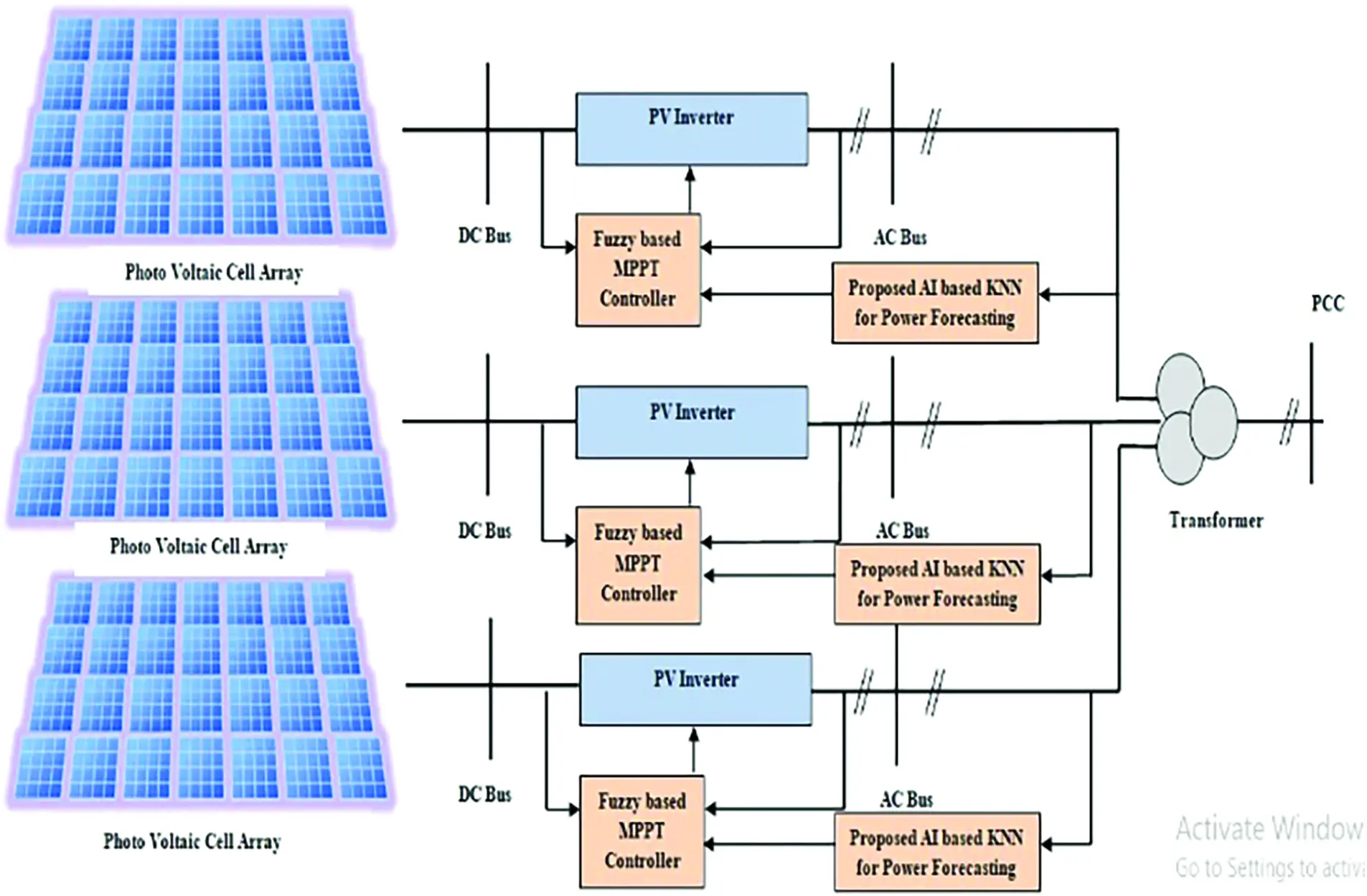Figure 1: Photovoltaic power generation using fuzzy based MPPT controller

3.1 PV Inverter

The PV inverter in the solar power generation system converts the variable Direct Current (DC) generated from the solar photovoltaic cells into Alternating Current (AC) at a utilization frequency. The converted alternating current can be employed for commercial and industrial applications using electric grid. The proposed solar photovoltaic cell is composed of cascaded multilevel inverter which accepts voltages at a minimum of three different levels and the full bridge topology inverter constructed with four switches is employed to synthesize the three level output voltage waveform. The three level output voltage obtained at the inverter is mathematically represented in Eq. (4).

Vo=Vo.1+Vo.2+Vo.3++Vo.n (4)

If the input dc voltage of all the solar PV sources is equal to the Vdc then the inverter is considered to be in symmetric level. In case of non-attainment of symmetrical level, the fuzzy system adjusts the photovoltaic cell in such a way to attain the symmetric level. The number of output voltage depends on the number of full bridges constructed in the inverter circuit and is represented as (2n + 1), where “n” is the number of bridges. The inverter output voltage of the proposed system is controlled by the fuzzy based controller which controls the position of the solar panel to obtain the maximum voltage from the solar PV systems.

3.2 Fuzzy Based MPPT Controller

Fig. 2 portrays the detailed process of the fuzzy logic controller. From the Fig. 2, the input signal is processed by the fuzzification process and allots a value to the input signals. The fuzzification process is followed by the inference mechanism to generate a clear data based on the set of rules followed in the inference mechanism. The inference mechanism is continued by the defuzzification process.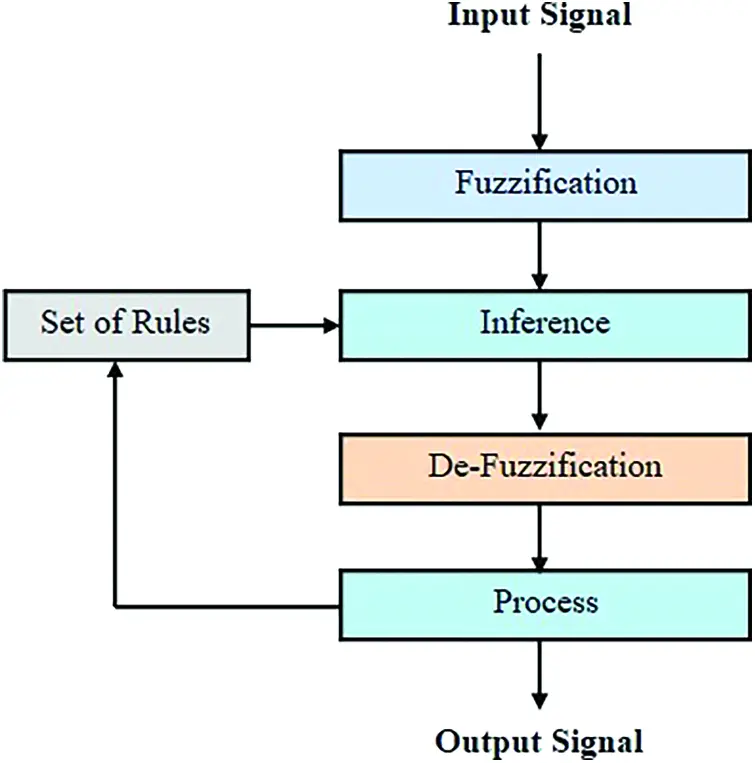Figure 2: Process of fuzzy based MPPT controller

The detailed process performed in the fuzzy based MPPT controller in the solar photovoltaic cell power generation depends on the fuzzification and de-fuzzification process as explained. The set of rules to be followed in fuzzy based MPPT controller in mentioned in Eqs. (10) and (11) respectively.

3.3 Fuzzification Process

The fuzzy controller depicted in Fig. 2 is driven by two inputs and one process output. The two input variables are mentioned as the Error and the Change Error which is mathematically expressed in Eqs. (5) and (6).

E(t)=P(t)P(t1)V(t)V(t1)=ΔPΔV (5)

EC=E(t)E(t1)=ΔE (6)

where, EC is the input variable change in Error, ΔP is the change in power generated and ΔE is the change in energy generated.

The input variable change of Error (EC) is defined on achieving the predefined condition of displacement of operating point towards the direction of Maximum Power Point. The output function increases with respective to the change in the duty cycle ΔD, whose value varies from negative range to the positive range of values. The output of this fuzzy based controller is fed as the input to the DC-DC converter in the solar photo voltaic cell power generation which is operated to drive the load at the constant voltage level. The accumulator placed in the PV system prepares to receive the value of duty cycle ΔD using the Eq. (6)

ΔD=D(t)D(t1) (7)

3.4 Inference Process

The inference process is performed on the basis of set of rules defined in the fuzzy based MPPT controlling process and the rule table for fuzzy based controlling process is illustrated in Fig. 3. In Fig. 3, the matrix is represented with entries depending on the values of the Error (E), the change in the duty ratio, and the change in the error values.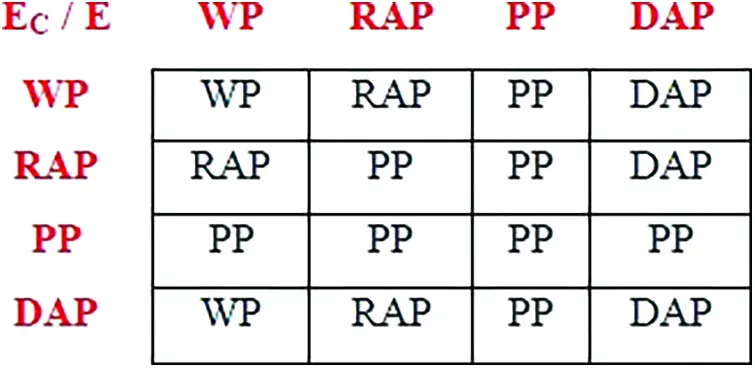Figure 3: Matrix of fuzzy associative function

The Fig. 3 provides the matrix of fuzzy associative function with 49 sets of rules to control the Maximum Power Point tracking process. The fuzzification process is performed at four defined scales of generated power namely: “WP” – Weak Power point, “PP” Peak Power point and the average power point is classified into two scales namely “RAP” – Rising Average Point and “DAP” – Decreasing Average Point. The rising average point is plotted when the successive power levels are in increasing manner while the Decreasing Average Point is plotted if the successive power points are in decreasing manner. The fuzzification process is performed by comparing the successive power points of any two solar photovoltaic cells. The rules for plotting the fuzzification table are:

•   WP - WP yields Weak Power point “WP”

•   WP - RAP yields Rising Average Point “RAP”

•   WP – PP yields Peak Power point “PP”

•   WP - DAP yields Decreasing Power Point “DAP”

•   RAP - RAP yields Peak Power point “PP”

The set of rules were defined from the feedback of the output process and the rows were represented by the change of Error (EC) while the columns were defined by the values of error (E).

3.5 De-Fuzzification Process

The de-fuzzification process is the core function of the fuzzy based MPPT controlling process, in which the output of the defuzzification process is fed to the Pulse Width modulation process to generate the pulse for driving the semiconductor MOSFET switch in the DC-DC converter. The defuzzification process is performed by two methodologies namely Centre of Area and Maximum Criterion Methodology. The Centre of Area method determines the controller output which acts as the centre of gravity for the previous processed set of fuzzy process. The cumulative fuzzy process is determined by the performing sampling process of the incoming data and is computed by the Eq. (8).

Δd=i=0nμ(Ii)Iij=0nμ(Ij) (8)

3.6 Fuzzy Based MPPT Controller

The proposed fuzzy based MPPT controller drives the DC-DC converter of the solar photovoltaic cell power generation system. The proposed method is portrayed in Fig. 4 and it accept input voltage, input current and load current to the array of Analog to Digital Converters. The array of ADC accepts the analog input and converts it to equivalent digital values. The array of MPPT controller composed of set of Maximum Power Point Controller and Derivate controllers. The input digital value is accepted by the derivative controller and the derivative control frequency is equivalent to the circuit switching frequency.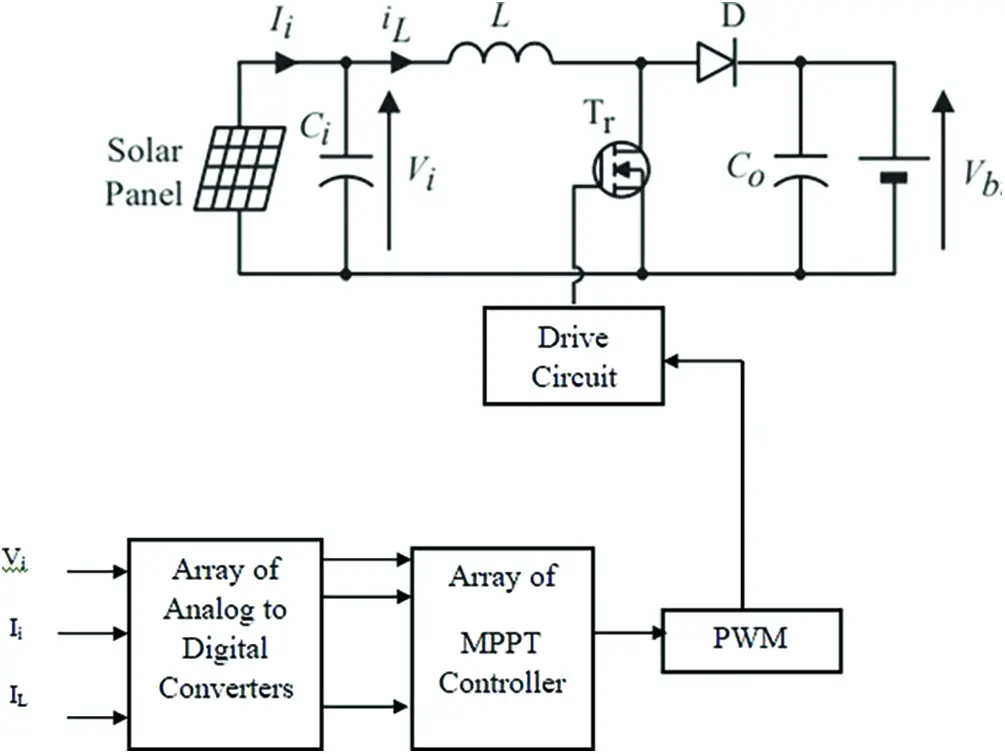Figure 4: Proposed fuzzy based MPPT controller

The MPPT controller which experiences maximum deviation will drive the Pulse Width Modulator (PWM) more effectively resulting in a high width output pulse given to the driver circuit. The width of the pulse generated by the Pulse Width Modulator (PWM) controls the MOSFET switch; the greater the deviation between the generated output and the MPPT point with generated more voltage (approximately 5 V) which drives the MOSFET to ON status. The lesser the difference generates reduced width of pulse (approximately less than 5 V) which is not sufficient to drive the MOSFET. The driver circuit accepts the pulses of all MPPT controllers and based on the width voltage, the driver circuit converts the MOSFET from switch OFF status to the switch ON status by driving the gate terminal. The MOSFET on switching ON, will allows the current to flow through from source to drain resulting in rotating of solar photovoltaic panel. This process is repeated until the MPPT controller produce voltage less than the threshold voltage such that the PWM output width will be minimum. The derivative control frequency is mathematically expressed in Eq. (9).

Dc(n)=KC[DiL(n1)DiL(n2)] (9)

where,

•   KC is the derivative coefficient

•   n represents the switching period

The input voltage and the input current are supplied to the fuzzy based MPPT controller to determine the power generated by the solar photovoltaic cells. The derivative control frequency DC(n) and the change in switching on frequency ΔDTON(n) controls the MPPT time period. The switching On time of the pulse width modulator (PWM) is determined by the ΔDTON(n) in the Differential Pulse Width Modulator. Artificial Intelligence in Solar power forecasting process: This section describes the forecasting process of solar power generation by the photovoltaic cell for the upcoming week based on the datasets collected from the present week and the data related to the weather condition. The different data collected as processed using the K-Nearest Neighbor algorithm of Artificial Intelligence which is diagrammatically described in Fig. 5.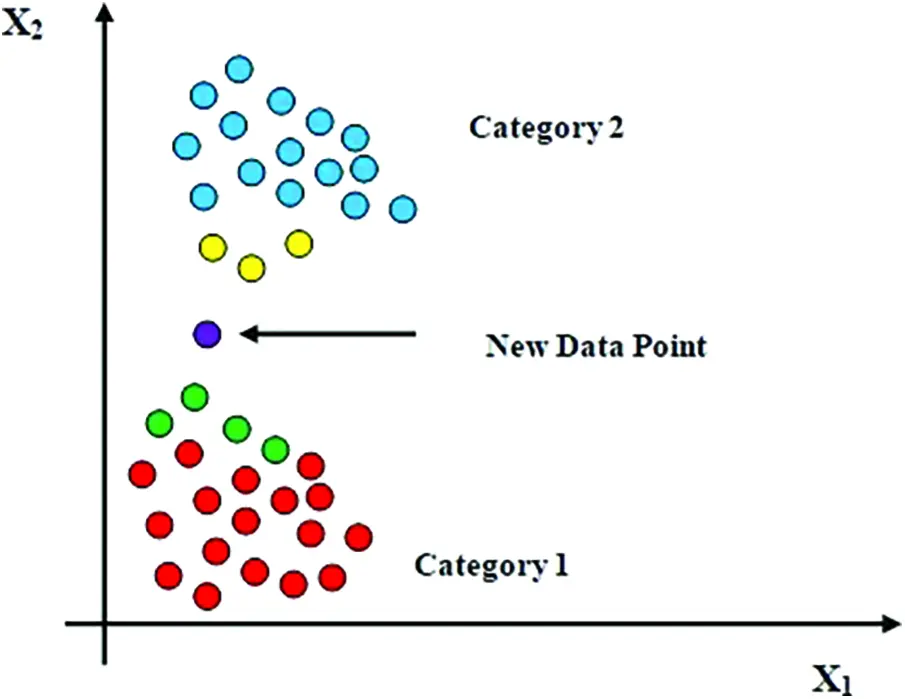Figure 5: K-nearest neighbor algorithm for artificial intelligence

The Fig. 5 illustrates the existence of two different types of data gathered from the photovoltaic cells and the weather report condition for the present week. The K-Nearest Neighbor algorithm relates these two data to determine the new data point which is highlighted in violet color. The new data point is derived from the four neighbor points from category 1 which is highlighted in green color and three neighbor data point in category 2 which is highlighted in yellow color. The block diagram representing the position of the K-Nearest Neighbor (KNN) algorithm in the proposed model of forecasting solar power generated by the photovoltaic cells is depicted in Fig. 6.Figure 6: KNN model in solar forecasting process

The Fig. 6 outlines the forecasting process of solar irradiation using proposed Artificial Intelligence algorithm of K-Nearest Neighbor. The K-Nearest Neighbor algorithm is the least parameter algorithm which is classified under supervised machine learning model. The KNN algorithm is executed by opting the suitable K value for the input data by selecting the right K value among many values. The reference model processed data and the KNN model processed data are compared to identify the error created in the forecasting process which is fed back to the KNN model through the Learning algorithm until the error becomes zero. The step by step and the detailed process performed in K Nearest Neighbor algorithm is illustrated in Tab. 1.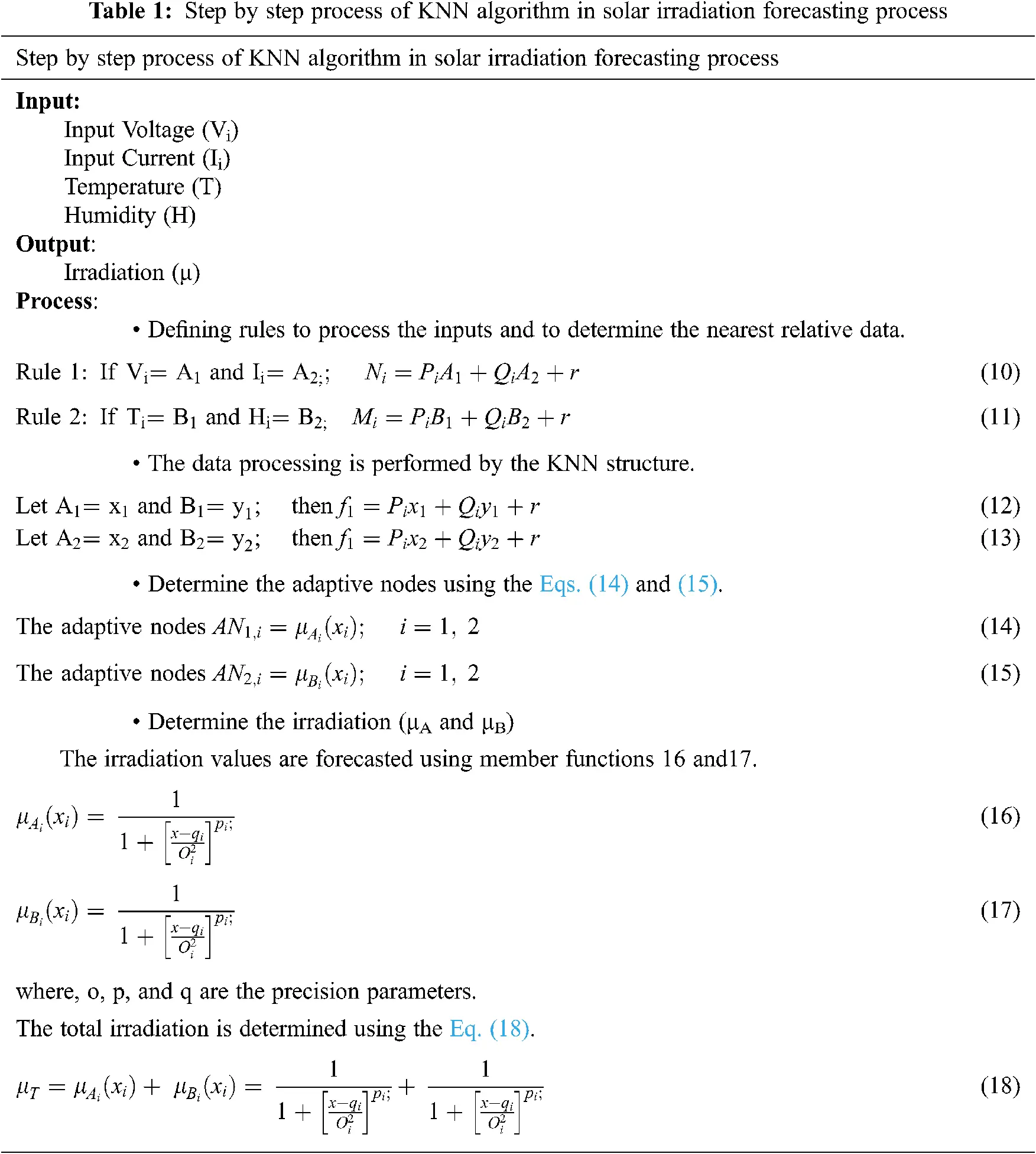The Eq. (17) illustrates the final forecasted value of the total irradiation produced by the solar photovoltaic cells. The “i” value is differed by the number of solar power grids present in the solar power plant. The proposed K Nearest Neighbor algorithm shall be modified and enhanced to determine the solar irradiation by performing validation of data and weighting of test data as described. The validity of the measured data is determined using the Eq. (19)

Vd=1Ki=1KS(label(xi),label(yi),label(AN1,i),label(AN1,i) (19)

The weighting of the test data is performed by employing the data validity in both training data and measured test data. The mathematical expression for determining the weightage of the test data is expressed in Eq. (20).

W(x,y)=xdE+0.5 (20)

The Artificial Intelligence incorporated in this proposed system is superior to the existing state of art technologies in such a way that it automatically generates the difference between the generated power and Maximum power point and adjusts the Solar Photovoltaic cells accordingly which is absent is existing methods.

4  Results and Discussion

The proposed methodology of forecasting the solar irradiation from the solar photovoltaic cells using K-Nearest Neighbor algorithm of Artificial Intelligence and the design of fuzzy based Maximum Power Point Tracking controller is designed and executed using MATLAB 2021a. The result section is two folded with the analysis of multiple waveforms in first fold followed by the quantitative analysis of the proposed method in the second fold.

The Fig. 7 depicts the output current generated by the solar photovoltaic cells. The output voltage and the output current produced by the solar photovoltaic cells depends on the quantity of the solar irradiation incidents on the photo voltaic cells. The current produced by the solar panel have fluctuations and produce a constant output voltage. The total grid current and voltage generated by the solar grid is portrayed in the Figs. 811 which shows constant fluctuations.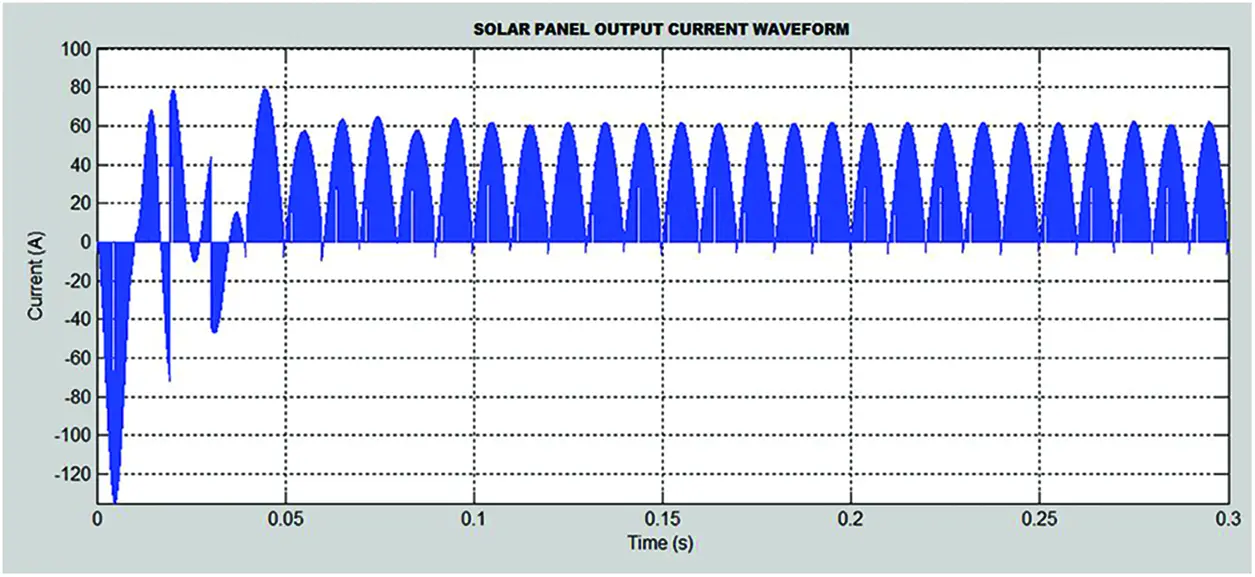Figure 7: Solar panel output current waveformFigure 8: Solar panel output voltage waveform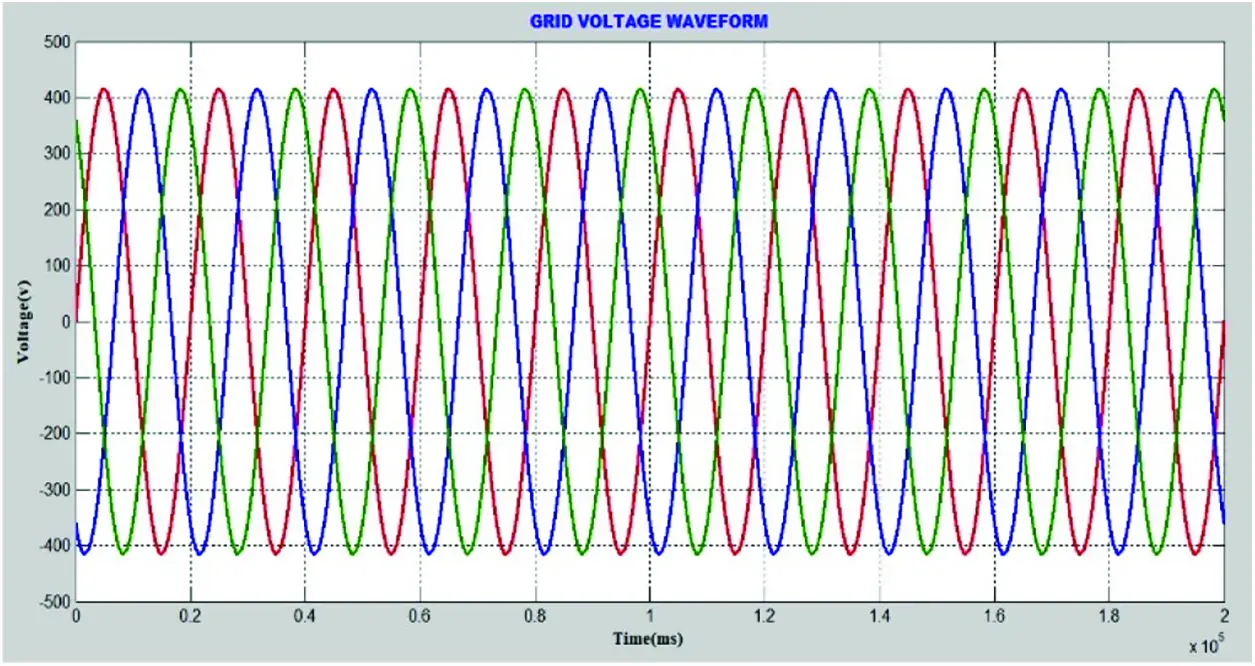Figure 9: Grid voltage waveform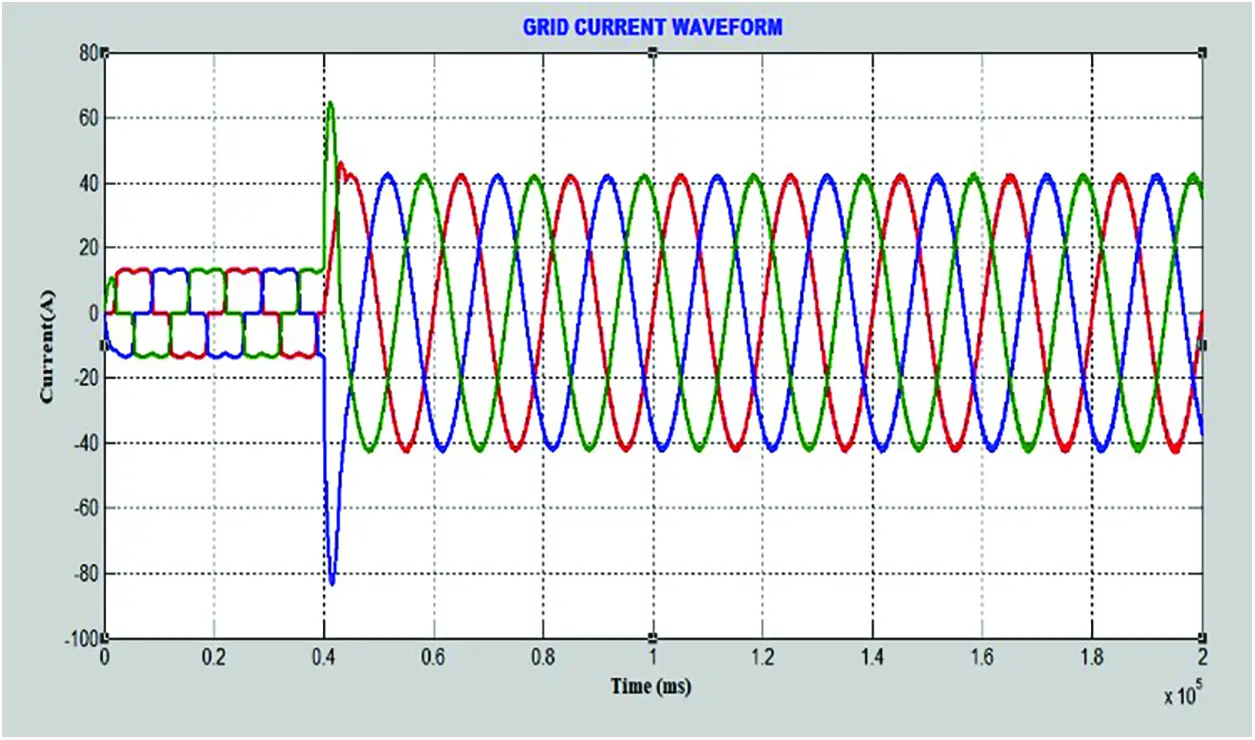Figure 10: Grid current waveform

The grid voltage and the grid current exhibits fluctuations at constant time intervals due to the variations in the power generated in individual solar photovoltaic cells. The accumulated voltage and current will experience constant fluctuations due to the variation of quantity of irradiation incident on the solar photovoltaic cells. The grid voltage and the current were analyzed for harmonic distortion and the Total Harmonic Distortion (THD) of the current generated by the solar photo voltaic cell is measured and is graphically represented in Fig. 11.Figure 11: Grid voltage and current waveform

The Total Harmonic Distortion in the solar grid current is measured by the considering the entire current component of the solar grid and it is defined as the ratio of the additive of entire current harmonic components to a fundamental frequency. The fundamental frequency considered in the proposed system is 50 Hz and the measured Total Harmonic Distortion is 1.01% shown in Fig. 12. The Total Harmonic distortion is reduced by using fuzzy based MPPT controller and the performance is compared with the conventional PI controller. The proposed fuzzy based MPPT controller is more efficient than the existing PI controller and the waveform and FFT representation of proposed fuzzy based controller and existing PI controller is depicted in Figs. 13 and 14.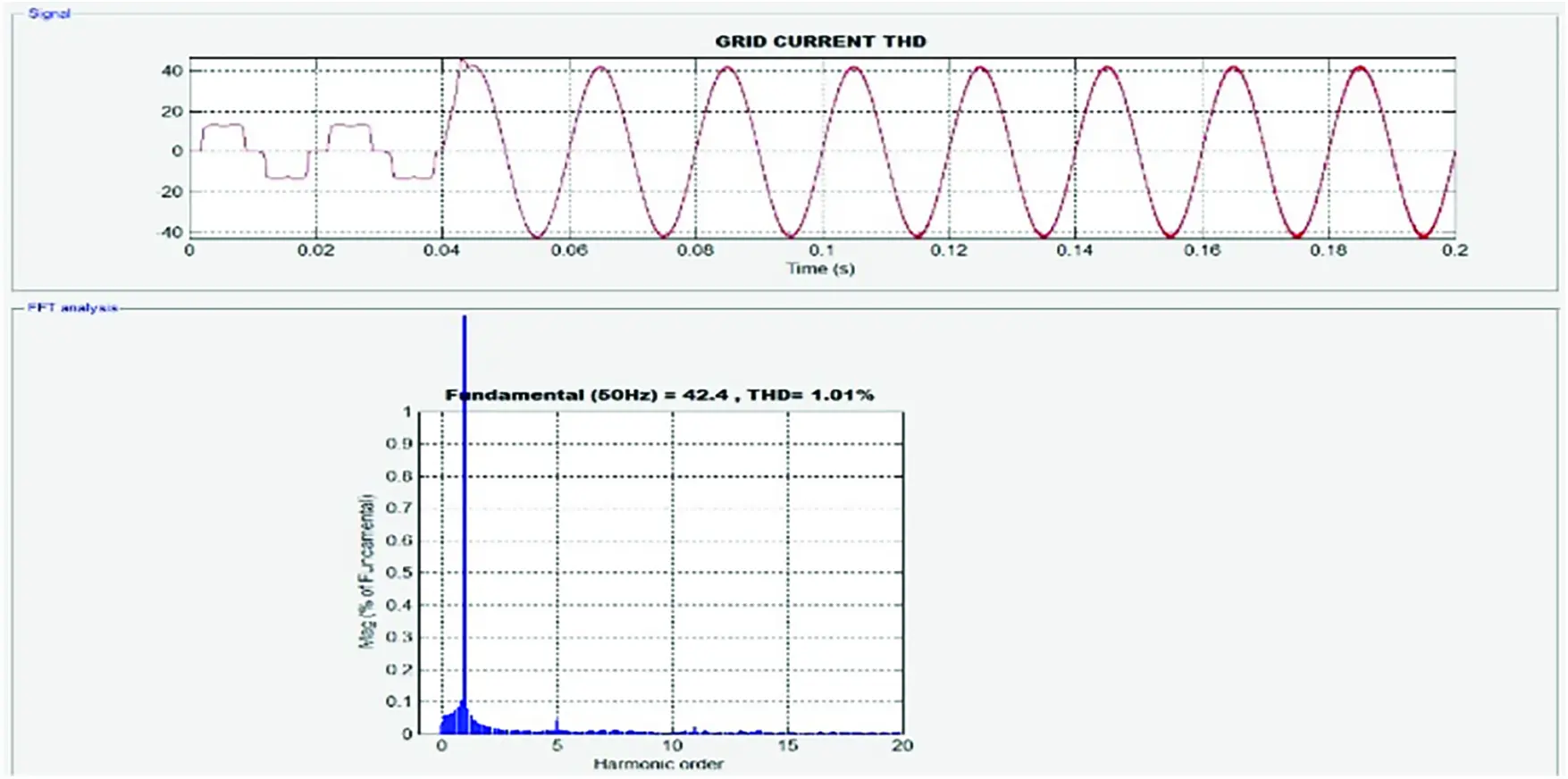Figure 12: Total harmonic distortion (THD) in solar grid current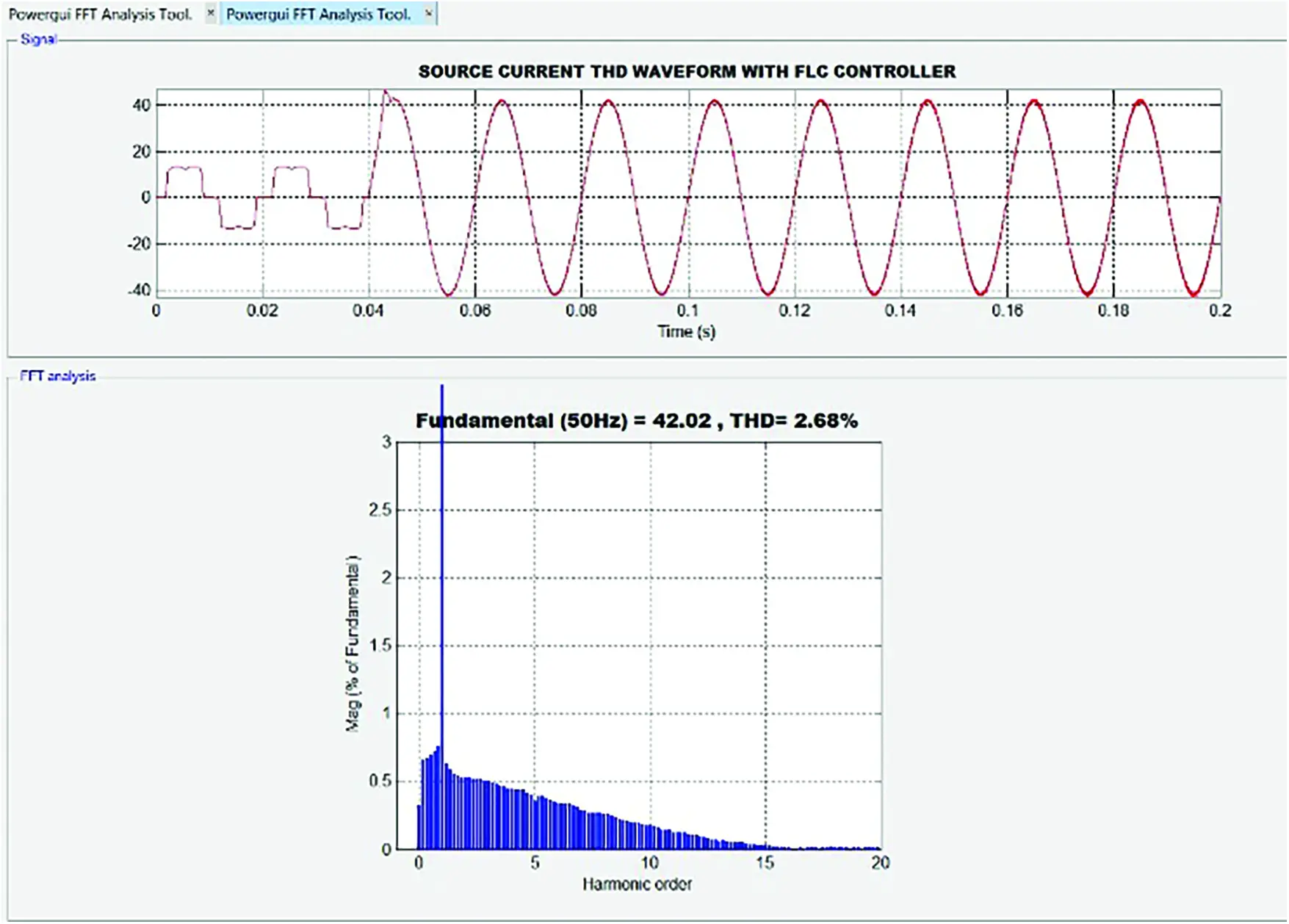Figure 13: Solar current THD waveform with fuzzy logic controllerFigure 14: Solar current THD waveform with PI controller

The comparison of fuzzy based controller and the PI controller is depicted in afore portrayed figures which yields that the proposed fuzzy controller possess a Total Harmonic Distortion of 2.68% while the existing PI controller exhibits a Total Harmonic Distortion of 3.79%. The output power encompassed of both real power and reactive power is measured after performing the fuzzy based MPPT controlling process and is diagrammatically displayed in Figs. 15 and 16.Figure 15: Real power waveform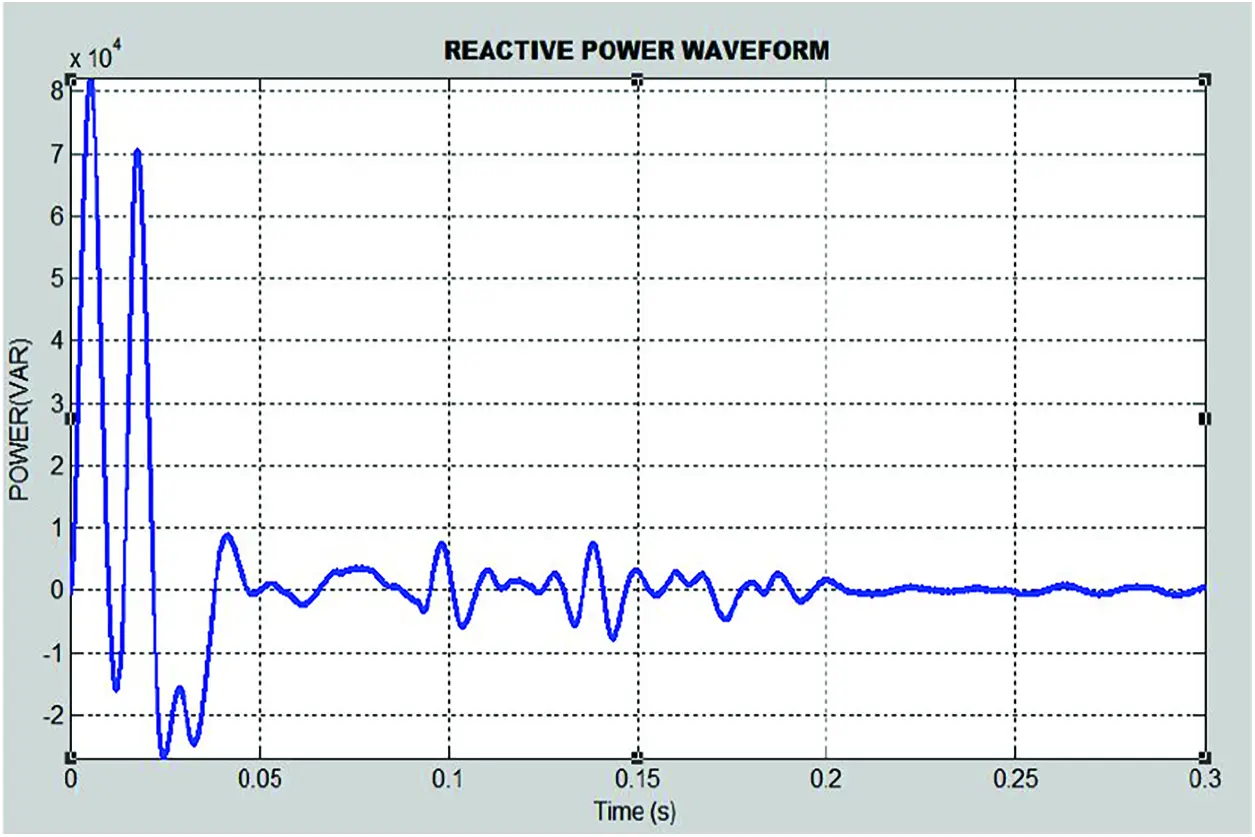Figure 16: Reactive power waveform

The Fig. 17 depicts the output voltage waveform with equal fluctuations among all the solar grid power panels. The quantitative analysis of the proposed method of Artificial Intelligence based solar irradiation forecasting method is performed on multiple statistical concepts like Mean Bias based Error (MBE), Mean Absolute Percentage Error (MAPE) and Root Mean Squared Error (RMSE). The listed statistical methods can be mathematically expressed as in Eqs. (21)(23).

MBE=1Ni=1N(x^(i)x(i)) (21)

MAPE=1Ni=1N|x^(i)x(i)x(i)| (22)

RMSE=1Ni=1N(x^(i)x(i)) (23)

where, the x(i) mentions the mean value of the measured data.Figure 17: Output voltage waveform

The proposed method of solar irradiation forecasting mechanism in solar power generation using photovoltaic cells has been designed in multiple models with reference to the three IEEE bus models like IEEE 14 bus system, 23 bus system, IEEE 30 bus system and IEEE 57 bus systems. The measurement and analysis of the aforementioned bus systems using the parameters defined in Eqs. (19)(21) are listed in Tab. 2. In the Tab. 2, the IEEE bus 14 system is considered as model 1, 23 bus system as model 2, 30 bus system as model 3 and finally 57 bus system as the model 4. IEEE 14 bus system is a simpler model comprises of 5 generators and 14 interconnection buses. The IEEE 23, 30 and 57 will be composed of 23, 30 and 57 number of buses along with generators in the circuit.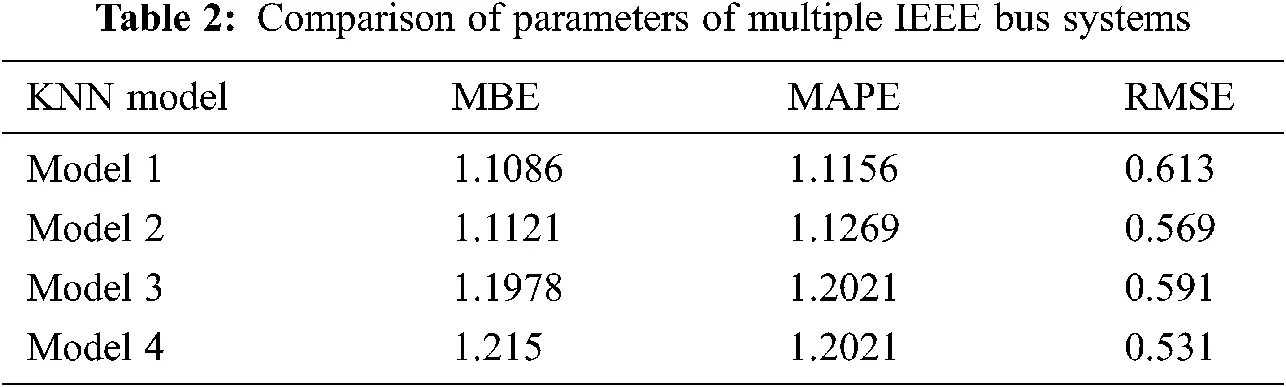The statistical analysis of the proposed methodology with different IEEE models has been analyzed and the mean RMSE value is identified to be 0.576% which is considered to be more efficient than the existing forecasting methodologies. The necessity of using different models for analysis of the proposed methodology is to test the proposed model against different complexion types of circuits. Here four different models with different level of complexity have been designated as Model 1 to Model 4 to analyze the performance metrics of proposed model.

The Tab. 3 explains the comparative analysis of the forecasting of the solar irradiation using Machine Learning algorithms, Deep Learning algorithms and the proposed K-Nearest Neighbor algorithm in Artificial Intelligence (AI) technique. The graphical analysis proves that the proposed methodology outperforms than the existing methodology with a Root Mean Square Error of 0.576%, thus providing high level accuracy in forecasting the solar irradiation in the solar photo voltaic cell power generation systems.5  Conclusion

The proposed methodology of employing K-Nearest Neighbor of Artificial Intelligence (AI) technique for forecasting the solar radiation and the fuzzy based Maximum Power Point Tracker controller is presented and is analyzed quantitatively. The proposed model outperforms well than the existing methodologies and provides an high level of accuracy with Root mean square error of 0.576% and thus provides an accuracy of above 99.424% which is considered to be the more efficient and accurate forecasting methodology. The analysis was performed for period of 6 months and with pre-defined data sets and using IEEE bus models for designing the solar power generation systems. Besides, the fuzzy based MPPT controller has been compared with the existing PI controller for the critical parameter of Total Harmonic Distortion (THD). The THD of the PI controller is 3.79% while the THD of the proposed fuzzy based MPPT controller is 1.01% which is considered to be more efficient.

Funding Statement: The authors received no specific funding for this study.

Conflicts of Interest: The authors declare that they have no conflicts of interest to report regarding the present study.

## References

1. R. M. Elavarasan, G. M. Shafiullah, S. Padmanaban, N. M. Kumar, A. Annam et al., “A comprehensive review on renewable energy development, challenges, and policies of leading Indian states with an international perspective,” IEEE Access, vol. 8, no. 20, pp. 74432–74457, 2020.
2. M. Opeyemi and A. Bello, “Path to sustainable energy consumption: The possibility of substituting renewable energy for non-renewable energy,” Energy, vol. 2, no. 228, pp. 120519–120531, 2021.
3. S. R. Salkuti, “Multi-objective based optimal scheduling of microgrid considering uncertainties,” in Int. Conf. on Cutting-Edge Technologies in Engineering, Uttar Pradesh, India, vol. 14, pp. 50–56, 2019.
4. K. Atluri, S. M. Hananya and B. Navothna, “Performance of rooftop solar pv system with crystalline solar cells,” in National Power Engineering Conf. (NPECMadurai, India, vol. 12, pp. 1–4, 2018.
5. C. singer and D. Matthews, “Dual use solar surfaces for local grids and net zero development,” in IEEE Electric Power and Energy Conf. (EPECEdmonton, Canada, vol. 9, pp. 1–5, 2020.
6. A. A. Edris, “Opportunities and challenges of integrating wind, solar and other distributed generation & energy storage: effects on and values for the grid,” in IEEE Power and Energy Society General Meeting, San Diego, CA, USA, vol. 12, pp. 1–4, 2012.
7. K. Kajiwara, N. Matsui and F. Kurokawa, “A new MPPT control for solar panel under bus voltage fluctuation,” in IEEE 6th Int. Conf. on Renewable Energy Research and Applications (ICRERASan Diego, CA, USA, vol. 9, pp. 1047–1050, 201
8. M. Z. Hassan, M. E. K. Ali, A. B. M. S. Ali and J. Kumar, “Forecasting day-ahead solar radiation using machine learning approach,” in Asia-Pacific World Congress on Computer Science and Engineering (APWC on CSEMana Island, Fiji, vol. 2, pp. 252–258, 2017.
9. M. Aslam, K. H. Seung, S. Jae Lee, J. M. Lee, S. Hong et al., “Long-term solar radiation forecasting using a deep learning approach-gRUs,” in IEEE 8th Int. Conf. on Advanced Power System Automation and Protection (APAPchina, vol. 13, pp. 917–920, 201
10. H. Suryoatmojo, R. Mardiyanto, D. C. Riawan, E. Setijadi, S. Anam et al., “Design of MPPT based fuzzy logic for solar-powered unmanned aerial vehicle application,” in Int. Conf. on Engineering, Applied Sciences, and Technology (ICEASTPhuket, Thailand, vol. 7, pp. 1–4, 2018.
11. K. Muthumayil, R. Karuppathal, T. Jayasankar, B. Aruna Devi, N. B. Prakash et al., “A big data analytical approach for prediction of cancer using modified k-nearest neighbour algorithm,” Journal of Medical Imaging and Health Informatics, vol. 11, no. 8, pp. 2184–2189, 2020.
12. S. Lyden and T. Ji, “A study into the impact of the choice of maximum power point tracking technique on the reliablilty of the power electronics interface for photovoltaic systems,” in Australasian Universities Power Engineering Conf. (AUPECHobart, Australia, vol. 2, pp. 1–5, 2020.
13. H. Liu, J. C. Gu and M. T. Yang, “A simplified lstm neural networks for one day-ahead solar power forecasting,” IEEE Access, vol. 9, pp. 17174–17195, 2021.
14. D. Mukherjee, S. Chakraborty, P. K. Guchhait and J. Bhunia, “Machine learning based solar power generation forecasting with and without mppt controller,” in Int. Conf. for Convergence in Engineering (ICCEKolkata, India, vol. 5, pp. 44–48, 2020.
15. H. Sangrody, N. Zhou and Z. Zhang, “Similarity-based models for day-ahead solar PV generation forecasting,” IEEE Access, vol. 8, pp. 104469–104478, 2020.
16. Z. Si, Y. Yu, M. Yang and P. Li, “Hybrid solar forecasting method using satellite visible images and modified convolutional neural networks,” IEEE Transactions on Industry Applications, vol. 57, no. 1, pp. 5–16, 2021.
17. K. Doubleday, S. Jascourt, W. Kleiber and B. M. Hodge, “Probabilistic solar power forecasting using Bayesian model averaging,” IEEE Transactions on Sustainable Energy, vol. 12, no. 1, pp. 325–337, 2021.
18. Z. Zhen, J. Liu, Z. Zhang, F. Wang, H. Chai et al., “Deep learning based surface irradiance mapping model for solar pv power forecasting using sky image,” IEEE Transactions on Industry Applications, vol. 56, no. 4, pp. 3385–3396, 2020.
19. R. Ramakrishna, A. Scaglione, V. Vittal, E. Dall'Anese and A. Bernstein, “A model for joint probabilistic forecast of solar photovoltaic power and outdoor temperature,” IEEE Transactions on Signal Processing, vol. 67, no. 24, pp. 6368–6383, 20
20. S. Córdova, H. Rudnick, Á. Lorca and V. Martínez, “An efficient forecasting-optimization scheme for the intraday unit commitment process under significant wind and solar power,” IEEE Transactions on Sustainable Energy, vol. 9, no. 4, pp. 1899–1909, 2018.
21. S. Lu and H. F. Hamann, “A methodology for quantifying reliability benefits from improved solar power forecasting in multi-timescale power system operations,” IEEE Transactions on Smart Grid, vol. 9, no. 6, pp. 6897–6908, 2018.
22. A. Tascikaraoglu, B. M. Sanandaji, G. Chicco, V. Cocina, F.Spertino et al., “Compressive spatio-temporal forecasting of meteorological quantities and photovoltaic power,” IEEE Transactions on Sustainable Energy, vol. 7, no. 3, pp. 1295–1305, 2016.
23. Z. E. Z. Laggoun, N. Khalile and H. Benalla, “A comparative study between DPC-SVM and PDPC-SVM,” in Int. Conf. on Advanced Electrical Engineering (ICAEEAlgiers, Algeria, vol. 12, pp. 1–5, 2019.
24. S. Sun and R. Huang, “An adaptive k-nearest neighbor algorithm,” in Seventh Int. Conf. on Fuzzy Systems and Knowledge Discovery, Yantai, China, vol. 12, pp. 91–94, 2010.This work is licensed under a Creative Commons Attribution 4.0 International License, which permits unrestricted use, distribution, and reproduction in any medium, provided the original work is properly cited.